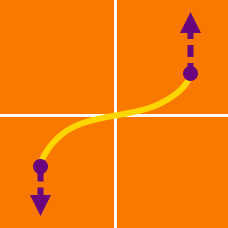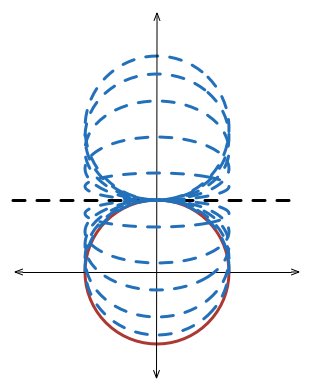Calculus

# Arc Length and Surface Area - Problem SolvingWhat is the area of the surface obtained by rotating the circle $x^2+y^2=15 ^2$ about the line $y=15 ?$

Hint: You can use $\int \frac{du}{\sqrt{a^2-u^2}}=\sin^{-1} \frac{u}{a}+C,\ a > 0.$

What is the length of the curve represented by the equation $\displaystyle x^{2/3}+y^{2/3}=1?$

What is the length of the curve $\displaystyle y=\int_{3}^{x} \sqrt{t^3-1} \,dt$ in the domain $1 \leq x \leq 4 ?$

What is the area of the surface obtained by rotating the loop of the curve $48y^2=x(16-x)^2$ about the $x$-axis?

A delivery drone flying at constant speed $15 \text{ m/s}$ and constant height $2700 \text{ m}$ toward a destination drops its goods. If the trajectory of the falling goods until it hits the ground can be described by the equation $y=2700-\frac{x^2}{75},$ where $x$ is the horizontal distance it travels and $y$ is its height above the ground, what is the distance (not horizontal displacement) traveled by the goods until it hits the ground?

Note: You can use $\displaystyle \int \sqrt{a^2+u^2}\,du=\frac{u}{2}\sqrt{a^2+u^2}+\frac{a^2}{2}\ln(u+\sqrt{a^2+u^2})+C.$

×